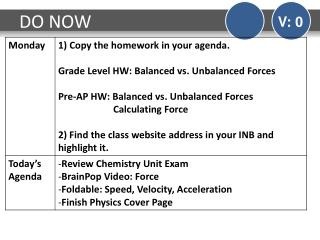DownloadDownload PresentationDO NOW

# DO NOW

Download Presentation## DO NOW

- - - - - - - - - - - - - - - - - - - - - - - - - - - E N D - - - - - - - - - - - - - - - - - - - - - - - - - - -
##### Presentation Transcript

1. DO NOW V: 0

2. TEKS

3. Review Chemistry Unit Exam V: 2 Review the Chemistry Unit Exam

4. BrainPop Video V: 0 BrainPop: Force

5. Foldable V: 0 Create a foldable for Speed, Velocity, and Acceleration.

6. Foldable V: 0

7. Foldable V: 0

8. Foldable V: 0

9. Foldable V: 0

10. Finish Physics Cover Page V: 2 Finish the cover page for Physics in your INB. Cover page must have: - the word “Physics” - Physics is the study of forces, motion, and energy!! - at least 4 pictures representing Physics - at least 4 different colors

11. DO NOW V: 0

12. TEKS

13. Force Foldable V: 0 Create a FORCE foldable by taping/gluing the handouts in your INB.

14. Force Foldable V: 0 What is force?

15. Force Foldable V: 0 What is force? A push or pull that can change the motion of an object.

16. Force Foldable V: 0 What 2 things does every force have?

17. Force Foldable V: 0 What 2 things does every force have? Force has a strength and direction.

18. Force Foldable V: 0 How is force measured?

19. Force Foldable V: 0 How is force measured? Force is measured in Newtons.

20. Force Foldable V: 0 Describe and give examples of balanced and unbalanced forces.

21. Net Force Practice Problems V: 0 When the arrows are pointing in the same direction…

22. Net Force Practice Problems V: 0 When the arrows are pointing in the same direction… ADD

23. Net Force Practice Problems V: 0 When the arrows are pointing in the same direction… ADD When the arrows are pointing in opposite directions…

24. Net Force Practice Problems V: 0 When the arrows are pointing in the same direction… ADD When the arrows are pointing in opposite directions… SUBTRACT

25. Net Force Practice Problems V: 0 Copy each of the practice problems in your INB and then answer the three questions. What is the net force? Are the forces balanced or unbalanced? Describe the motion of the object. (None, Accelerates Right, Accelerates Left) 10 N 20 N

26. Net Force Practice Problems V: 0 Copy each of the practice problems in your INB and then answer the three questions. What is the net force? Are the forces balanced or unbalanced? Describe the motion of the object. (None, Accelerates Right, Accelerates Left) 30 N 30 N

27. Net Force Practice Problems V: 0 Copy each of the practice problems in your INB and then answer the three questions. What is the net force? Are the forces balanced or unbalanced? Describe the motion of the object. (None, Accelerates Right, Accelerates Left) 20 N 50 N

28. Net Force Practice Problems V: 0 Copy each of the practice problems in your INB and then answer the three questions. What is the net force? Are the forces balanced or unbalanced? Describe the motion of the object. (None, Accelerates Right, Accelerates Left) 40 N 40 N

29. Net Force Practice Problems V: 0 Copy each of the practice problems in your INB and then answer the three questions. What is the net force? Are the forces balanced or unbalanced? Describe the motion of the object. (None, Accelerates Right, Accelerates Left) 30 N 15 N

30. Exit Ticket V: 0 Complete the Exit Ticket

31. DO NOW V: 0 25 N 15 N

32. TEKS

33. Tug-of-War V: 0 Create the table below in your INB.

34. Tug-of-War Simulator V: 0 Simulator Click on “Run Now”

35. Tug-of-War V: 0 Describe an example where there was an unbalanced force. How did you know it was unbalanced?

36. Tug-of-War V: 0 Draw a picture of a trial where the forces were unbalanced with arrows showing the direction of the forces.

37. Tug-of-War V: 0 Draw a picture of a trial where the forces were balanced with arrows showing the direction of the forces.

38. Reading & Cornell Notes V: 0 Read: Force and Motion Cornell Notes

40. Force and Motion video V: 0 Video

41. Exit Ticket V: 0 Draw a picture in your INB of yourself performing your favorite hobby. Use arrows and words to describe and label the forces acting on your and/or objects in your drawing.

42. Bubble Gum Lab V: 2 Follow the instructions on the handout to complete the Bubble Gum Lab.

43. DO NOW V: 0

44. TEKS